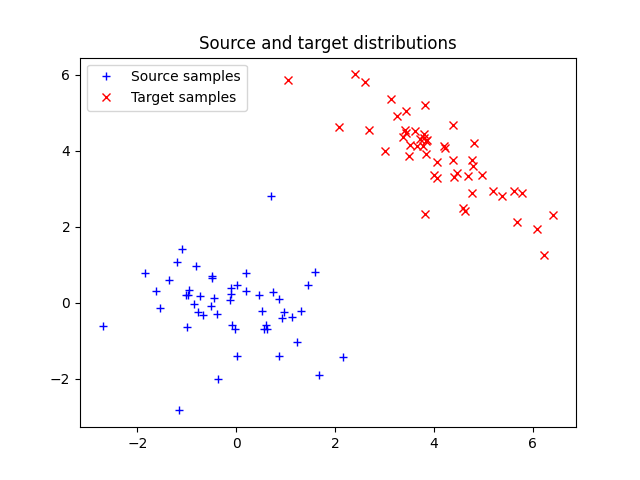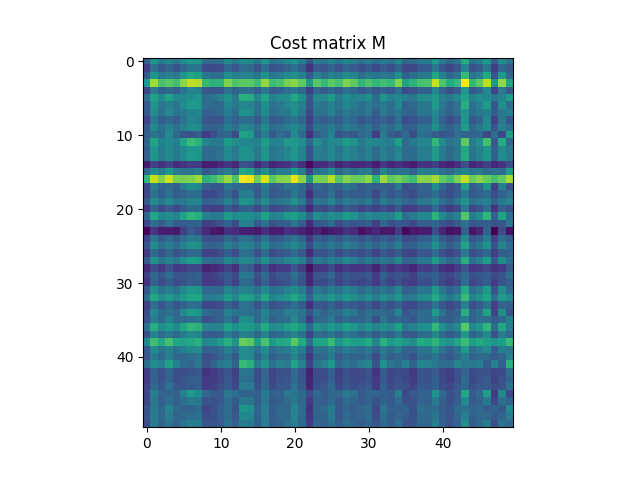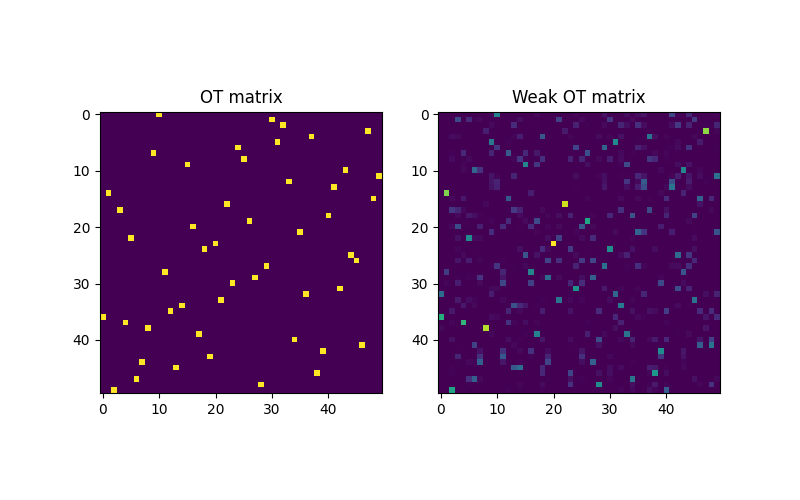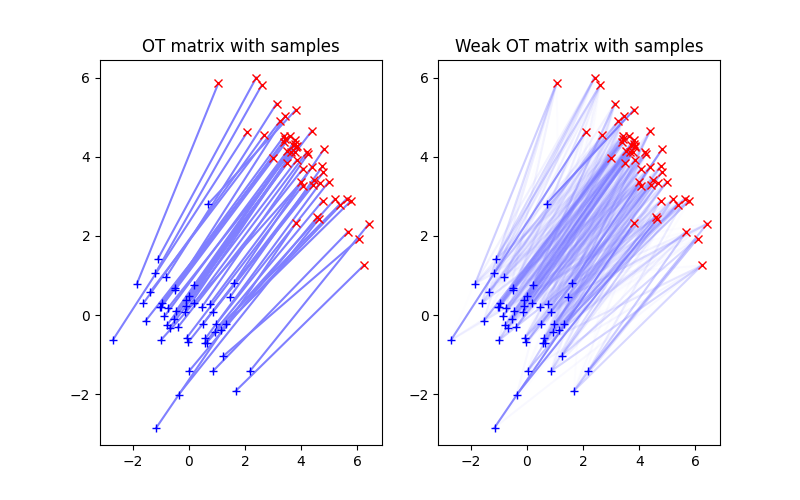# Weak Optimal Transport VS exact Optimal Transport

Illustration of 2D optimal transport between distributions that are weighted sum of diracs. The OT matrix is plotted with the samples.

```# Author: Remi Flamary <remi.flamary@polytechnique.edu>
#

# sphinx_gallery_thumbnail_number = 4

import numpy as np
import matplotlib.pylab as pl
import ot
import ot.plot
```

## Generate data an plot it

```n = 50  # nb samples

mu_s = np.array([0, 0])
cov_s = np.array([[1, 0], [0, 1]])

mu_t = np.array([4, 4])
cov_t = np.array([[1, -.8], [-.8, 1]])

xs = ot.datasets.make_2D_samples_gauss(n, mu_s, cov_s)
xt = ot.datasets.make_2D_samples_gauss(n, mu_t, cov_t)

a, b = ot.unif(n), ot.unif(n)  # uniform distribution on samples

# loss matrix
M = ot.dist(xs, xt)
M /= M.max()
```
```pl.figure(1)
pl.plot(xs[:, 0], xs[:, 1], '+b', label='Source samples')
pl.plot(xt[:, 0], xt[:, 1], 'xr', label='Target samples')
pl.legend(loc=0)
pl.title('Source and target distributions')

pl.figure(2)
pl.imshow(M, interpolation='nearest')
pl.title('Cost matrix M')
```
••```Text(0.5, 1.0, 'Cost matrix M')
```

## Compute Weak OT and exact OT solutions

```G0 = ot.emd(a, b, M)
```
```Gweak = ot.weak_optimal_transport(xs, xt, a, b)
```

## Plot weak OT and exact OT solutions

```pl.figure(3, (8, 5))

pl.subplot(1, 2, 1)
pl.imshow(G0, interpolation='nearest')
pl.title('OT matrix')

pl.subplot(1, 2, 2)
pl.imshow(Gweak, interpolation='nearest')
pl.title('Weak OT matrix')

pl.figure(4, (8, 5))

pl.subplot(1, 2, 1)
ot.plot.plot2D_samples_mat(xs, xt, G0, c=[.5, .5, 1])
pl.plot(xs[:, 0], xs[:, 1], '+b', label='Source samples')
pl.plot(xt[:, 0], xt[:, 1], 'xr', label='Target samples')
pl.title('OT matrix with samples')

pl.subplot(1, 2, 2)
ot.plot.plot2D_samples_mat(xs, xt, Gweak, c=[.5, .5, 1])
pl.plot(xs[:, 0], xs[:, 1], '+b', label='Source samples')
pl.plot(xt[:, 0], xt[:, 1], 'xr', label='Target samples')
pl.title('Weak OT matrix with samples')
```
••```Text(0.5, 1.0, 'Weak OT matrix with samples')
```

Total running time of the script: ( 0 minutes 2.554 seconds)

Gallery generated by Sphinx-Gallery